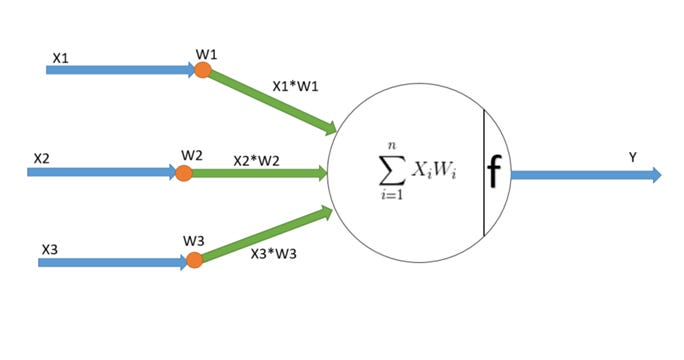Function of the neuron

In the previous blog, we discussed the need for an artificial brain and the basic architecture of it. Now in this blog, it will be very interesting to see how a neuron function works, what’s the math behind it, how do I simulate it with a simple example. I will try to answer all these questions.

The math behind the neuron function

I denoted all the neurons with the letter “f” which is for me doing some function. The function can be different based on the types of neuron you want to choose for solving a complex problem. But let’s start with something which is a linear equation f(x)=Wx+B. You can read more about the math function the blog written by my friend Paresh

X = Input of the neuron

W = Synaptic weight while connecting with another neuron. The synaptic weight is the strength of the connection between synapsis of the one neuron to the dendrite of another neuron. The weight amplifies the input signal thus the dendrite of another neuron carries the multi

B = Bias for the neuron

Y = Output from the neuron

The core function of the neuron:

Summation (∑): Execute the summation of all the input multiplied by the weights plus bias.

Threshold (f): Threshold function which will fire the neuron. Also known as the Activation function. An example of the activation function is the Step function which says with the output of summation reaches the threshold value defined; the neuron will fire with the threshold value otherwise will be zero. The choice of activation depends upon the problem either regression, classification

How does a neuron learn?

The learning process of a neuron involves reading the input X and trying to find the answer Y by tuning the value W (Synaptic weight) which is the base of all supervised learning process. In the supervised learning, we provide the network with training data having input and output. The real purpose of the learning process is to find the approx. Weight value which will fit all the values with less error margin. The popular algorithm for this is called “Backpropagation”. The backpropagation algorithm was originally introduced in the 1970s, but its importance wasn’t fully appreciated until a famous 1986 paper by David Rumelhart, Geoffrey Hinton, and Ronald Williams. That paper describes several neural networks where backpropagation works far faster than earlier approaches to learning, making it possible to use neural nets to solve problems which had previously been insoluble. Today, the backpropagation algorithm is the workhorse of learning in neural networks.

I will simulate a simple learning process for an AND gate. Below are the input and output table of an AND gate:

X1X2Y000010100111

Above is the neural diagram with two input taking all the combination of 0 or 1. Let’s initialise the synaptic weight to some value say “0..1”, and since we will manually optimise the network to find the solution best, I am going to use a learning rate of 0.1 which means after each iteration of training process I will update the weights by incrementing by 0.1, train again until we find the final weight which solves above network.

Iteration 1 (First pass)

Apply the summation to the first record: (0 x 0.1) + (0 x 0.1) = 0

Pass the summation to the step function with a threshold value to 1: if X < 1 then 0 else 1 which in this case output is 0

The output of the step function is compared with the actual value.

Similarly, apply to the other three rows as well for weight value 0.1.

X1X2SumStep FuncY00000010.100100.100110.201

You see that the final result is 75% accurate which need to be improved. Let’s do the learning process by incrementing the weight by adding 0.1

Iteration 2(Second Pass)

Apply the summation to the first record: (0 x 0.2) + (0 x 0.2) = 0

Pass the summation to the step function with a threshold value to 1: if X < 1 then 0 else 1 which in this case output to 0

Similarly, apply to the other three rows as well for weight value of 0.2

X1X2SumStep FuncY00000010.200100.200110.401

Still not improved and sits with 75%. Continue the training process.

Iteration 3

X1X2SumStep FuncY00000010.300100.300110.601

.

.

.

Iteration 6

Similarly, apply to other three rows as well for weight value 0.6

X1X2SumStep FuncY00000010.600100.600111.211

The accuracy reached 100% with 6th iteration.

Well, the above process is the simulation of the backpropagation algorithm which made the network learn and come up with the final weights:

In the next blog, I will try to represent the above smilation with C# code which will be more interesting for the coders. This is just the start and we need to understand basics before actually going deeper into designing much complex network. Stay tuned!!!!

Source: Deep Learning on Medium